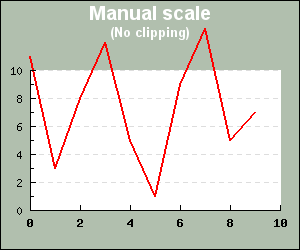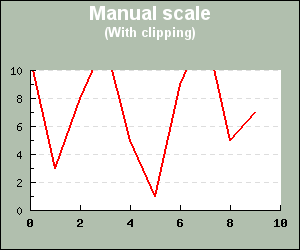## 14.3. Adjusting the look and feel of the plot area

The graph area can be divided in two parts.

1. The margin area

2. The plot area

These areas can have individual background colors as well as edges of user settable size and color. In addition it is possible to use an image as background with different formatting options.

### 14.3.1. Plot area and margin areas

The plot area is where the plot is drawn and the margin area is everything outside. Methods that affect the format of the plot area are

1. `Graph::SetMargin() `, This will adjust the size (and position) of the plot area

2. `Graph::SetBox(\$aDrawPlotFrame=true,\$aPlotFrameColor=array(0,0,0),\$aPlotFrameWeight=1)`, This will adjust tyhe format of the edge around the plot area. By default this is disabled.

In a similar way the margin areas peroperties can be adjusted with the methods

1. `Graph::SetMargin()` , same as above

2. `Graph::SetMarginColor(\$aColor)`

3. `Graph::SetFram(\$aDrawImgFrame=true,\$aImgFrameColor=array(0,0,0),\$aImgFrameWeight=1)`

4. `Graph::SetFrameBevel(\$aDepth=3,\$aBorder=false,\$aBorderColor='black',\$aColor1='white@0.4',\$aColor2='darkgray@0.4',\$aFlg=true)`

### 14.3.2. Clipping to the plot area

When auto scaling is used no data points will ever be outside the plot area since the scaling will make sure it covers the dynamic range of the data. However, when setting the scale manually there is always the risk that some data points are outside the specified scale. This could then lead to the result shown in Figure 14.13 To avoid this situation it is possible to enable clipping to the plot region by calling the method

• `Graph::SetClipping(\$aFlg)`

This will "cut away" all parts of the plot that would otherwise be stroked outside the plot area.Figure 14.14 shows the result after clipping has been enabled.

 Figure 14.13. Original plot without clipping `(clipping_ex1.php)`Figure 14.14. Plot with clipping enabled `(clipping_ex2.php)`### Note

By default clipping is disabled since it will slightly increase the CPU load and the time to generate the graphs.